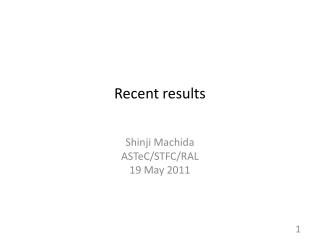# Recent results - PowerPoint PPT PresentationDownload PresentationRecent results

Recent results
Download Presentation## Recent results

- - - - - - - - - - - - - - - - - - - - - - - - - - - E N D - - - - - - - - - - - - - - - - - - - - - - - - - - -
##### Presentation Transcript

1. Recent results Shinji Machida ASTeC/STFC/RAL 19 May 2011

2. Orbital period (Time of Flight) measurement • Data fits nicely as a parabola curve • Empty circles are measurement, filled circles are after including correction of velocity.

3. Orbit without acceleration • Each cell has one BPM between QD and QF. • 42 cells correspond to 1 turn. • Figures below show 10 turns results with 12.0 MeV/c equivalent momentum. horizontal vertical

4. Orbit position vs momentum • Calculate average and standard deviation of orbit measurement in the previous page. • Standard deviation is mostly determined by COD as well as initial mismatch of orbit.

5. Cell tune without acceleration • NAFF applied to consecutive 21 cells gives instantaneous cell tune over 10 turns. horizontal vertical

6. Cell tune vs momentum • Cell tune at each equivalent momentum calculated with NAFF shows momentum dependent tune.

7. Orbit and (hori) tune with acceleration • With rf, a beam is accelerated and horizontal orbit position and H and V cell tunes changes as a function of momentum. • Horizontal position (left) and horizontal tune (right).

8. Serpentine channel acceleration (1) • By comparing phase information, longitudinal phase space can be reconstructed. Momentum determined by H orbit Momentum determined by H tune

9. Serpentine channel acceleration (2) • By comparing phase information, longitudinal phase space can be reconstructed. Momentum determined by V tune

10. Beam centre oscillations • No significant effects of tune crossing. • Centroid oscillation amplitude does not grow much.

11. letter • Results will be submitted to a journal letter shortly.